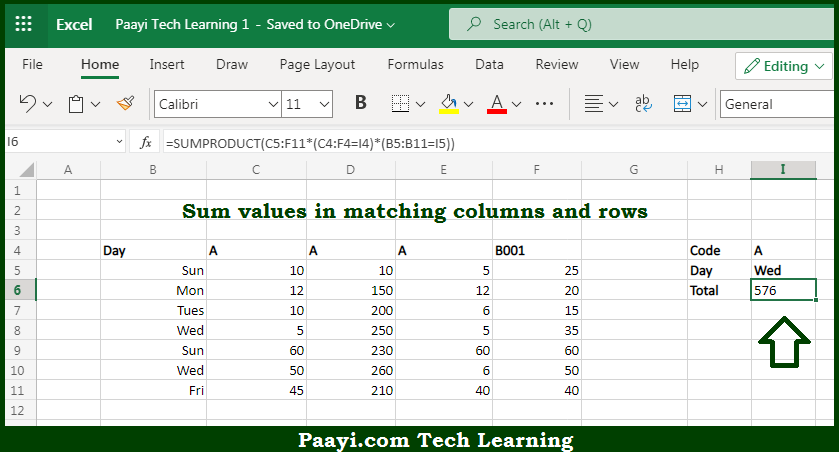# Learn How to SUM Matching Columns and Rows in Microsoft Excel

Written by | 0 Comments | 628 Views

In this article, you will learn how to SUM various things in Microsoft Excel using a single/combination(s) of functions. You will also know how to SUM Matching Columns and Rows and see the generic formula.

SUM Matching Columns and Rows in Microsoft Excel

The main purpose of this formula is to sum values in matching columns and rows. Here we will learn how to sum matching columns including rows in the workbook in Microsoft Excel. That implies, with the help of a formula based on the SUMPRODUCT function you can able sum values in matching columns and rows. So, with the help of this formula, you can able to sum matching columns including rows in the workbook in Microsoft Excel.

General Formula to SUM Matching Columns and Rows

=SUMPRODUCT(data*(range1=criteria1)*(range2=criteria2))

The Explanation for the SUM Matching Columns and RowsSo we know that with the help of the given formula above you can able to sum values in matching columns and rows. Here we will learn how to sum matching columns including rows in the workbook in Microsoft Excel. As we know that the SUMPRODUCT function effectively handles arrays, moreover it also does not require control shift enter. In the example provided here, we are multiplying all values in the named range data by two expressions that filter out values, not of interest. The first expression applies a filter based on code and the second expression filters on the day. So, with the help of this formula, you can able to sum matching columns including rows in the workbook in Microsoft Excel.